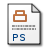# Numerical Modelling of Eulerian Two-Phase Gas-Solid Flow

Hudson, Justin and Harris, David (2006) Numerical Modelling of Eulerian Two-Phase Gas-Solid Flow. [MIMS Preprint]PDF JuH-1Dnd0.pdf Download (1MB)Postscript JuH-1Dnd0.ps Download (2MB)

## Abstract

This paper investigates the numerical solution of the equations governing two-phase flow of a solid granular material dispersed in a gas. We consider two different models in both of which the dispersed and continuous phases are treated as continua. An Eulerian description of the flow is adopted. Four different formulations of the two models are derived and a high resolution scheme is presented to obtain numerical solutions of the equations in each of the formulations. We investigate whether the chosen numerical scheme is suitable for the equations governing the models and use the numerical results to obtain quantitative and qualitative insight into the predictions of each of the models. Three test cases, new to the literature, are considered, and the numerical results compared.

Item Type: MIMS Preprint MSC 2010, the AMS's Mathematics Subject Classification > 35 Partial differential equationsMSC 2010, the AMS's Mathematics Subject Classification > 65 Numerical analysisMSC 2010, the AMS's Mathematics Subject Classification > 76 Fluid mechanics Dr Justin Hudson 26 Jan 2006 08 Nov 2017 18:18 http://eprints.maths.manchester.ac.uk/id/eprint/151View Item